Go through the Math in Focus Grade 1 Workbook Answer Key Chapter 19 Practice 4 Adding and Subtracting Money to finish your assignments.

Example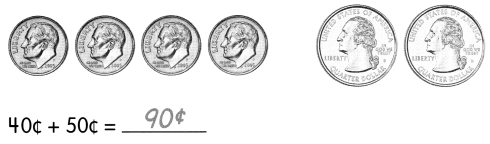Question 1.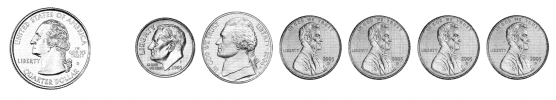25¢ + 19¢ = ___________¢
Explanation:
1 quarter + (1 dime + 1 nickel + 4 pennies)
25 + (10 + 5 + 1 + 1 + 1 + 1)= 44¢
25¢ + 19¢ =  44¢

Question 2.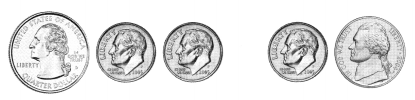45¢ + 15¢ = _____________¢
Explanation:
(1 quarter+ 2 dimes) + (1 dime + 1 nickel)
(25 + 10 + 10) + (10 + 5) = 60¢
45¢ + 15¢ =  60¢

Question 3.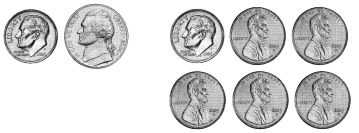15¢ + 15¢ = _____________¢
Explanation:
(1 dime + 1 nickel ) + (1 dime + 5 pennies)
(10 + 5 ) + (10 + 1 + 1 + 1 + 1 + 1)= 30¢
15¢ + 15¢ =  30¢

Question 4.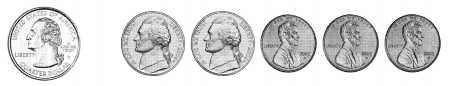25¢ + 13¢ = _____________¢
Explanation:
1 quarter + (2 nickels + 3 pennies)
25 + (5 + 5 + 1 + 1 + 1) = 38¢
25¢ + 13¢ = 38¢

Example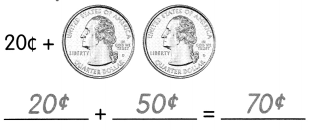Question 5.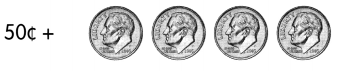___________ + __________ = ____________
Explanation:
50¢ + 4 dimes
1 dime = 10¢
50 + 10 + 10 + 10 + 10 = 90¢

Question 6.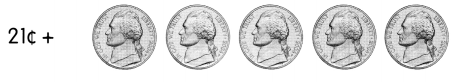___________ + __________ = ____________
Explanation:
21¢ + 5 nickels
1 nickel = 5¢
21 + 5 + 5 + 5 + 5 + 5 = 46¢

Question 7.___________ + __________ = ____________
Explanation:
8¢ + (2 dimes + 2 nickels)
1 dime = 10¢
1 nickel = 5¢
8 + (10 + 10 + 5 + 5)
8 + 30 = 38¢

Question 8.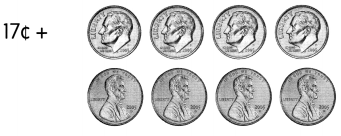___________ + __________ = ____________
Explanation:
17¢ + (4 dimes + 4 pennies)
1 dime = 10¢
1 penny = 1¢
17 + (10 + 10 + 10 + 10 +1 + 1+ 1+ 1)
17 + 44 = 61¢

Question 9.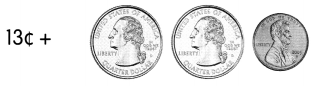___________ + __________ = ____________
Explanation:
13¢ + (2 quarters + 1 penny)
1 quarter = 25¢
1 penny = 1 ¢
13 + (25 + 25 + 1)
13 + 51 = 64¢

Question 10.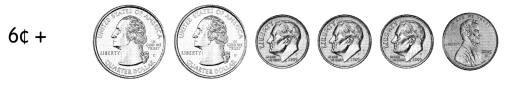___________ + __________ = ____________
Explanation:
6¢ + (2 quarters + 3 dimes + 1 penny)
1 quarter = 25¢
1 dime = 10¢
1 penny = 1¢
6 + (25 + 25 + 10 + 10 + 10 + 1)
6 + 81 = 87¢

Question 11.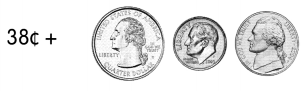___________ + __________ = ____________
Explanation:
38¢ + (1 quarter + 1 dime + 1 nickel)
1 quarter = 25¢
1 dime = 10¢
1 penny = 1¢
38 + (25 + 10 + 5)
38¢ + 40¢ = 78¢

The Art Club made cards to sell.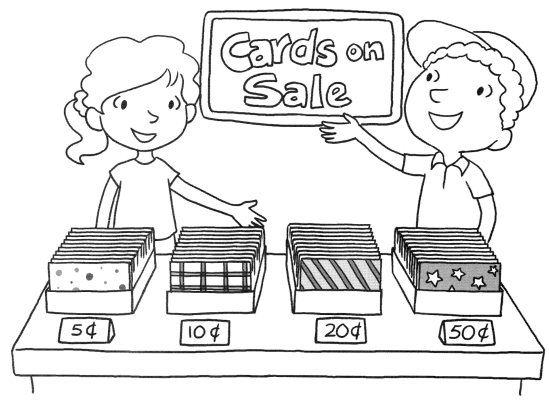Fill in the blanks.

Example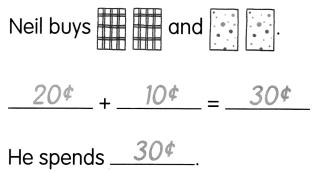Question 12.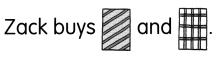___________ + __________ = ____________
He spends ___________
Explanation:
Zack buys 20¢ and 10¢ cards
Total amount he spends = 20¢ + 10¢ = 30¢

Question 13.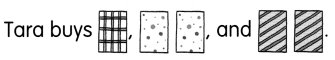___________ + __________ + __________ = ____________
She spends ___________.
Explanation:
Tara buys cards of 10¢ , 5¢, 5¢, 20¢, 20¢
Total amount she spends,
10¢ + 5¢ + 5¢ + 20¢ + 20¢ = 60¢

Question 14.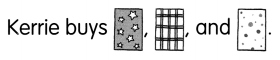___________ + __________ + __________ = ____________
She spends ___________.
Explanation:
Kerrie buys cards of 50¢ + 10¢ + 5¢
Total amount she spends,
50¢ + 10¢ + 5¢ = 65¢

Question 15.
How much do Zack and Neil spend in all?
___________ + __________ = ____________
They spend ___________ in all.
Explanation:
Zack has 30¢ and Neil has 30¢
Total amount they spend in all
30¢ + 30¢ = 60¢

Subtract.

Question 16.
55¢ – 20¢ = ______________
Explanation:
By subtracting small amount 20¢ from big amount 55¢
55¢ – 20¢ = 35¢

Question 17.
45¢ – 15¢ = ______________
Explanation:
By subtracting small amount 15¢ from big amount 45¢
45¢ – 15¢ = 30¢

Question 18.
60¢ – 5¢ = ______________
Explanation:
By subtracting small amount 15¢ from big amount 60¢
60¢ – 15¢ = 45¢

Question 19.
99¢ – 35¢ = _____________
Explanation:
By subtracting small amount 35¢ from big amount 99¢
99¢ – 35¢ = 64¢

Subtract

Example
50¢ from 60¢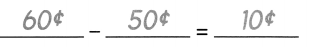Question 20.
35¢ from 50¢
__________ – ___________ = ____________
Explanation:
By subtracting small amount 35¢ from big amount 50¢
50¢ – 35¢ = 15¢

Question 21.
25¢ from 70¢
__________ – ___________ = ____________
Explanation:
By subtracting small amount 25¢ from big amount 70¢
70¢ – 25¢ = 45¢

Question 22.
60¢ from 90¢
__________ – ___________ = ____________
Explanation:
By subtracting small amount 60¢ from big amount 90¢
90¢ – 60¢ = 30¢

Question 23.
35¢ from 95¢
__________ – ___________ = ____________
Explanation:
By subtracting small amount 35¢ from big amount 95¢
95¢ – 35¢ = 60¢

Complete.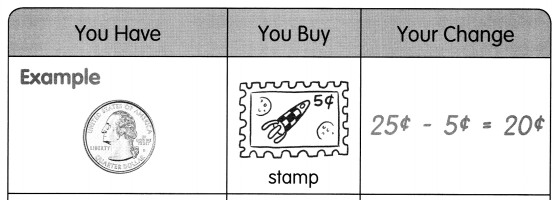Question 24.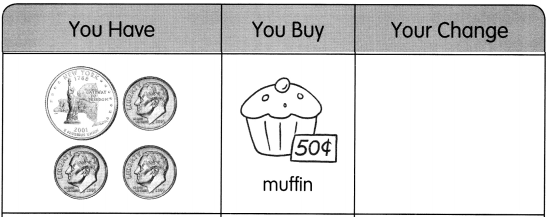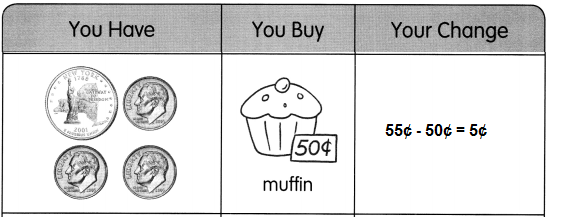Explanation:
1 quarter + 3 dimes
1 quarter = 25¢
1 dime = 10¢
25¢ + 10¢ + 10¢ + 10¢ = 55¢
55¢ – 50¢ = 5¢

Question 25.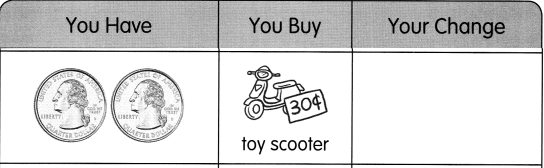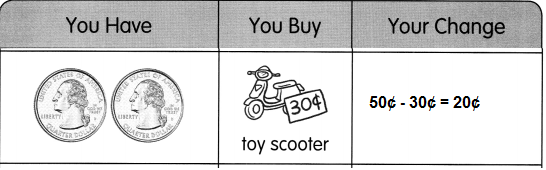Explanation:
2 quarters = 50¢
25¢ +25¢ = 50¢
50¢ – 30¢ = 20¢

Question 26.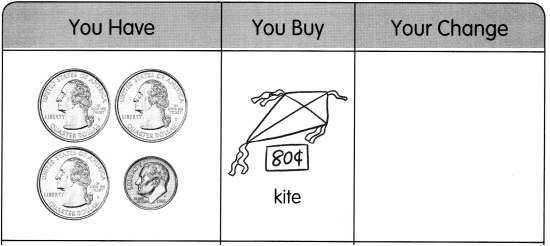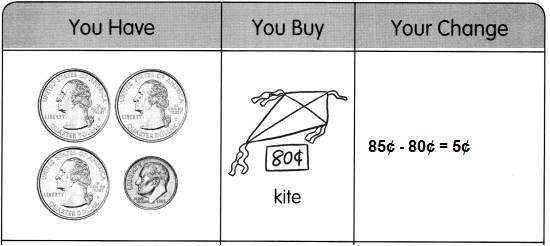Explanation:
3 quarters + 1 dime
1 quarter = 25¢
1 dime = 10¢
25¢ + 25¢ + 25¢ + 10¢ = 85¢
85¢ – 80¢ = 5¢

Question 27.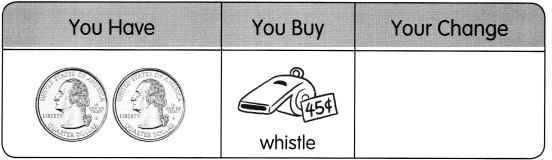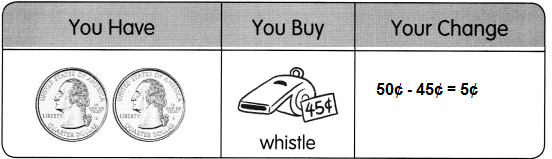Explanation:
2 quarters
25¢ + 25¢ = 50¢
50¢ – 45¢ = 5¢

Solve.

Example
Adam buys a pen and a doll. How much does he spend in all?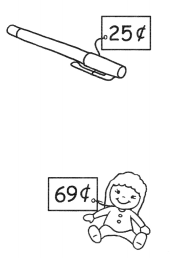25¢ + 69¢ = 94¢

Question 28.
Sherry buys a ball. She pays with three quarters. How much change does she get?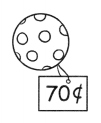She gets __________ in change.
Explanation:
Sherry buys a ball with three quarters.
Cost of ball = 70¢
Total change she gets
25¢ + 25¢ + 25¢ = 75¢
75¢ – 70¢ = 5¢

Solve.

Question 29.
Keri has 90¢. She buys a paper bag. How much does she have left?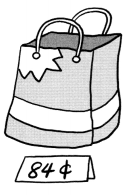She has __________ left.
Explanation:
Keri has 90¢.
Cost of paper bag 84¢
Total change she got
90¢ – 84¢ = 6¢

Question 30.
After buying a toy bicycle, Pat has 9¢ left. How much did he have at first?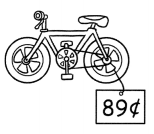Explanation:
Cost of toy cycle is 89¢
After buying a toy bicycle, Pat has 9¢ left.
Total amount he have at first
89¢ + 9¢ = 98¢

Question 31.
Andrew buys a pen. Eve buys a doll. How much less does Andrew pay than Eve?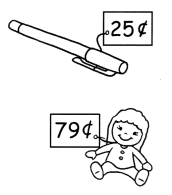Andrew pays _______ less than Eve.
Explanation:
Cost of pen is 25¢
Cost of doll 79¢
How much less does Andrew pay than Eve
79¢ – 25¢ = 54¢

Solve.

Question 32.
Derrick has 32¢. He wants to buy a toy bicycle. How much more does he need?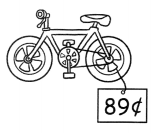Derrick needs __________ more.
Explanation:
Derrick has 32¢.
He wants to buy a toy bicycle.
Cost of toy cycle is 89¢
Total amount does he need
89¢ – 32¢ = 57¢

Question 33.
How much more is the pencil than the eraser?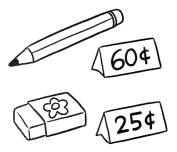The pencil is ________ more than the eraser.
Explanation:
Cost of pencil = 60¢
Cost of eraser = 25¢
How much more is the pencil than the eraser
60¢ -25¢ =35¢

Question 34.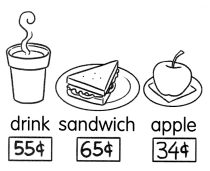He buys the ____________ and the ____________.
The sandwich and the apple
Explanation:
Cost of the sandwich  = 65¢
Cost of the apple = 34¢
Total amount Brad spend on both
65¢ +34¢ = 99¢

Gary and Fina are at the cafeteria.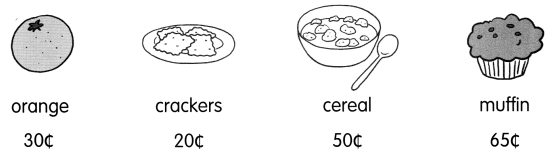Fill in the blanks.

Question 35.
Gary buys a bowl of cereal and crackers. How much does he spend in all? ____________
Explanation:
Cost of 1 bowl of cereal 50¢
Cost of crackers 20¢
Total amount Gary spend in all
50¢ +20¢ = 70¢

Question 36.
Gary uses 50¢ to buy an orange. How much change does he get? ____________
Explanation:
Gary uses 50¢ to buy an orange
Cost of orange 30¢
Total change he got after purchasing the orange
50¢ – 30¢ = 20¢

Question 37.
Fina uses two quarters to buy an orange. How much change does she get? ____________
20¢
Explanation:
Fina uses two quarters to buy an orange
25¢+ 25¢ = 50¢
Cost of 1 orange is 30¢
Total change he got after purchasing the orange
50¢ – 30¢ = 20¢

Question 38.
Gary buys a muffin and has 25¢ left. How much did he have at first? ____________
Answer: $1 Explanation: Gary buys a muffin and has 25¢ left. Cost of muffin 65¢ How much did he have at first 65¢ + 25¢ = 100¢ =$1

Question 39.
Fina has 3 dimes. She buys her food and has 10¢ left. What does she buy? _____________